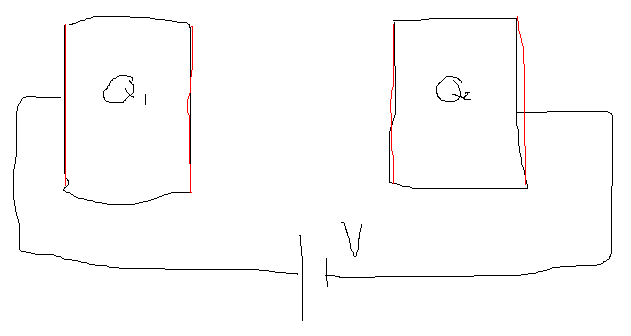# 2 charged conductors form a capacitor

feynman1
2 separate big conductors initially charged Q1 and Q2. Then connect them in a circuit with a battery of emf V. The charges Q1 and Q2 will go to the 4 surfaces (marked red). All the 4 surfaces have an area A. Suppose the 2 conductors form an ideal parallel plate capacitor and the wires in the circuit have no resistence. How to determine the charges on the 4 red surfaces?Homework Helper
Faculty looking for tips of designing PhD studentship interviews.
Is this the same user ?

Re this thread: do Q1 and Q2 have opposite signs ?

••Homework Helper
Gold Member
What makes you think that the final charge distribution is any different from what you get if you connect the two plates to the battery when there is no initial charge on them? Remember, the job of the battery is to maintain a constant potential difference V across its terminals. It will do just that in either case by adding/removing charges from the plates as needed.

feynman1
Is this the same user ?

Re this thread: do Q1 and Q2 have opposite signs ?
Q1 and Q2 might have the same or opposite signs

Homework Helper
2022 Award
There will be a net charge of Q1+Q2 distrbuted on the entire circuit and a charge Q=±VC separated on the capacitor plates where C is the Capacitance of the plates.
The details depend upon the exact geometry. To determine it you solve (or approximately solve) Maxwell's equations. Is there a point to this?

feynman1
There will be a net charge of Q1+Q2 distrbuted on the entire circuit and a charge Q=±VC separated on the capacitor plates where C is the Capacitance of the plates.
The details depend upon the exact geometry. To determine it you solve (or approximately solve) Maxwell's equations. Is there a point to this?
The plates are infinitely large. Let's care about the 4 red surfaces only. The geometry is already idealized, so we don't want to solve maxwell.

Homework Helper
2022 Award
If the plates are infinitely large,they will hold an infinite charge at any voltage . I think you need to specify your problem.

•feynman1
If the plates are infinitely large,they will hold an infinite charge at any voltage . I think you need to specify your problem.
Q1 and Q2 are amounts of charge, not density.

Homework Helper
2022 Award
To maintain V the battery will need to supply infinite charge ±∞. Doesn't matter otherwise.

feynman1
What makes you think that the final charge distribution is any different from what you get if you connect the two plates to the battery when there is no initial charge on them? Remember, the job of the battery is to maintain a constant potential difference V across its terminals. It will do just that in either case by adding/removing charges from the plates as needed.
The 2 outward facing sufaces will hold charges? But they should disappear due to the ideal wires (resistence=0)?

Homework Helper
2022 Award
Are your capacitors infinite??You need to specify the problem.

Homework Helper
Gold Member
The 2 outward facing sufaces will hold charges? But they should disappear due to the ideal wires (resistence=0)?
I never said or implied that the two outward surfaces hold charges. Furthermore the resistance of the wires has nothing to do with this. Once the battery is connected and the charges are redistributed, the left plate and the wire connected to it all the way to the positive terminal of the battery is an equipotential. Similarly, the right plate and wire is an equipotential.

•hutchphd
Homework Helper
Gold Member
Are your capacitors infinite??You need to specify the problem.
The plates cannot be mathematically infinite else there will be a potential difference between two points at infinity. They are "physically" infinite in the sense that the measure for their size (radius if circular, sides if rectangular) is much larger than the separation between them. In this limit the electric field between the plates can be considered uniform without fringing at the ends. That's the ideal parallel plate capacitor that OP specified in post #1.

Homework Helper
2022 Award
And the whole circuit could carry an arbitrary additional charge from say an ungrounded lab tech. It would be distributed all over the place. Of course the capacitor cannot be infinite..but you are correct he did specify "ideal" in the beginning and switched to infinite later.
The ideal air capacitor will carry surface charge densitie$$\sigma =\pm\epsilon_0\frac V {separation}$$ on the opposing faces.

..

feynman1
I never said or implied that the two outward surfaces hold charges. Furthermore the resistance of the wires has nothing to do with this. Once the battery is connected and the charges are redistributed, the left plate and the wire connected to it all the way to the positive terminal of the battery is an equipotential. Similarly, the right plate and wire is an equipotential.
So the 2 outward surfaces hold no charge? Then the total charge on the 2 inward surfaces=0, total charge isn't conserved any more (initially Q1+Q2)?